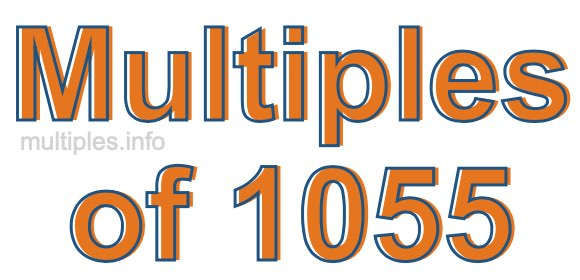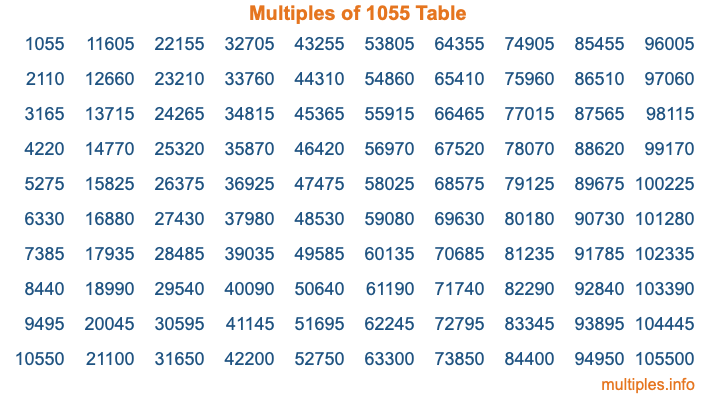Multiples of 1055Welcome to the Multiples of 1055 page. Here we will first teach you everything you will ever need to know about the multiples of 1055, and then give you a study guide summary of everything we taught you to make sure you remember it all. Use this page to look up facts and learn information about the multiples of 1055. This page will make you a multiples of one thousand fifty-five expert!

Definition of Multiples of 1055
Multiples of 1055 are all the numbers that when divided by 1055 equal an integer. Each of the multiples of 1055 are called a multiple. A multiple of 1055 is created by multiplying 1055 by an integer.

Therefore, to create a list of multiples of 1055, you start with 1 multiplied by 1055, then 2 multiplied by 1055, then 3 multiplied by 1055, and so on for as long as you want. Thus, the list of the first five multiples of 1055 is 1055, 2110, 3165, 4220, and 5275. To see a larger list of multiples of 1055, see the printable image of Multiples of 1055 further down on this page. We also have a category where you can choose any nth multiple of 1055.

Multiples of 1055 Checker
The Multiples of 1055 Checker below checks to see if any number of your choice is a multiple of 1055. In other words, it checks to see if there is any number (integer) that when multiplied by 1055 will equal your number. To do that, we divide your number by 1055. If the the quotient is an integer, then your number is a multiple of 1055.

Is  a multiple of 1055?

Least Common Multiple of 1055 and ...
A Least Common Multiple (LCM) is the lowest multiple that two or more numbers have in common. This is also called the smallest common multiple or lowest common multiple and is useful to know when you are adding our subtracting fractions. Enter one or more numbers below (1055 is already entered) to find the LCM.

Check out our LCM Calculator if you need more details about the Least Common Multiple or if you need the LCM for different numbers for adding and subtraction fractions.

nth Multiple of 1055
As we stated above, 1055 is the first multiple of 1055, 2110 is the second multiple of 1055, 3165 is the third multiple of 1055, and so on. Enter a number below to find the nth multiple of 1055.

th multiple of 1055

Multiples of 1055 vs Factors of 1055
1055 is a multiple of 1055 and a factor of 1055, but that is where the similarities end. All postive multiples of 1055 are 1055 or greater than 1055. All positive factors of 1055 are 1055 or less than 1055.

Below is the beginning list of multiples of 1055 and the factors of 1055 so you can compare:

Multiples of 1055: 1055, 2110, 3165, 4220, 5275, etc.

Factors of 1055: 1, 5, 211, 1055

As you can see, the multiples of 1055 are all the numbers that you can divide by 1055 to get a whole number. The factors of 1055, on the other hand, are all the whole numbers that you can multiply by another whole number to get 1055.

It's also interesting to note that if a number (x) is a factor of 1055, then 1055 will also be a multiple of that number (x).

Multiples of 1055 vs Divisors of 1055
The divisors of 1055 are all the integers that 1055 can be divided by evenly. Below is a list of the divisors of 1055.

Divisors of 1055: 1, 5, 211, 1055

The interesting thing to note here is that if you take any multiple of 1055 and divide it by a divisor of 1055, you will see that the quotient is an integer.

Multiples of 1055 Table
Below is an image of the first 100 multiples of 1055 in a table. The table is in chronological order, column by column. The first column has the first ten multiples of 1055, the second column has the next ten multiples of 1055, and so on.The Multiples of 1055 Table is also referred to as the 1055 Times Table or Times Table of 1055. You are welcome to print out our table for your studies.

Negative Multiples of 1055
Although not often discussed or needed in math, it is worth mentioning that you can make a list of negative multiples of 1055 by multiplying 1055 by -1, then by -2, then by -3, and so on, to get the following list of negative multiples of 1055:

-1055, -2110, -3165, -4220, -5275, etc.

Multiples of 1055 Summary
Below is a summary of important Multiples of 1055 facts that we have discussed on this page. To retain the knowledge on this page, we recommend that you read through the summary and explain to yourself or a study partner why they hold true.

There are an infinite number of multiples of 1055.

A multiple of 1055 divided by 1055 will equal a whole number.

1055 divided by a factor of 1055 equals a divisor of 1055.

The nth multiple of 1055 is n times 1055.

The largest factor of 1055 is equal to the first positive multiple of 1055.

1055 is a multiple of every factor of 1055.

1055 is a multiple of 1055.

A multiple of 1055 divided by a divisor of 1055 equals an integer.

1055 divided by a divisor of 1055 equals a factor of 1055.

Any integer times 1055 will equal a multiple of 1055.

Multiples of a Number
Here you can get the multiples of another number, all with the same attention to detail as we did for multiples of 1055 on this page.

Multiples of
Multiples of 1056
Did you find our page about multiples of one thousand fifty-five educational? Do you want more knowledge? Check out the multiples of the next number on our list!